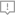﻿ 系统集成项目管理工程师案例分析每日一练试题（2021/1/11） - 每日一练 - 信管网

【说明】【问题1】（7分）
（1）请给出该项目初步进度计划的关键路径和工期。
（2）该项目进度计划需要压缩多少天才能满足工期要求？可能需要压缩的活动都有哪些？
（3）若项目组将B和H均压缩至30天，是否可满足工期要求？压缩后项目的关键路径有多少条？关键路径上的活动是什么？
【问题2】（9分）【问题3】（6分）

【问题4】（3分）

1.（1）初步进度关键路径为bgi，公司为120天。＜br＞（2）需要压缩20天满足工期要求，可能需要压缩的活动有abdgih。＜br＞（3）可以满足工期要求。＜br＞压缩后关键路径有一条，关键路径活动为adf。＜br＞2.a  活动：pv=180×20=3600  ev=180x20=3600＜br＞   b活动：pv=2×220×30=13200  ev=2×2×220=13200＜br＞活动c：pv=150×6=900   ev=0＜br＞活动d：pv=2×240×20=9600  ev=2×240×20=9600＜br＞活动e：pv=180×10=1800    ev=0＜br＞活动g：pv=2×200×10=4000    ev=2×200×10=4000＜br＞活动h：pv=0   ev=0＜br＞活动i：pv=0   ev=0＜br＞3.40天晚：pv=3600＋13200+900+9600+1800+4000+0=33100＜br＞ev=3600+13200+0+9600+0+4000＋0=30400＜br＞ac=40000＜br＞cv=ev-ac=30400-40000=-9600＜br＞sv=ev-pv=30400-33100=-2700＜br＞cpi=ev/ac=30400/40000=0.76＜br＞spi=ev/pv=30400/33100=0.92＜br＞当前绩效信息：cpi＜1，spi＜1，cpi＜0，spi＜0。＜br＞所以当前项目进度滞后，成本超支，效率低下。＜br＞采取措施：控制成本，减少人员成本，加大培训：加快进度，加班或赶工，并行施工，快速跟进；提高效率，改善工艺和技术。＜br＞4，bac=3600+13200+900+19200+1800+16000+8000+9000=71700＜br＞etc=bac-ev=71700-30400=41300＜br＞eac=ac＋etc=40000+41300=81300＜br＞＜br＞温馨提示：因考试政策、内容不断变化与调整，信管网网站提供的以上信息仅供参考，如有异议，请以权威部门公布的内容为准！

 发表评论  查看完整评论

### 相关内容10年专注，信管教育专注者，信管网优势介绍免费试听系统集成项目管理工程师课程全国前50名高分学员访谈：董丽(174)、李思...系统集成项目管理工程师高端班培训课程资深讲师60小时系统直播课程系统集成项目管理工程师历年真题与答案高级信息系统项目管理师通关培训课程系统规划与管理师培训课程（自有资料+直播）软题库：软考在线题库、支持手机答题